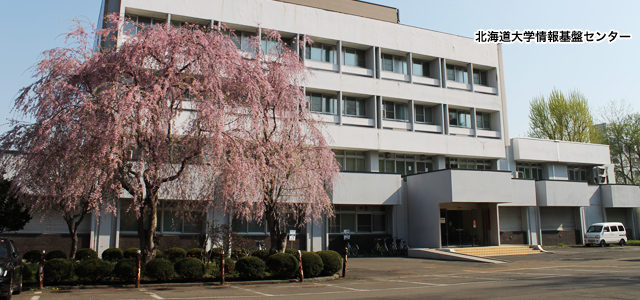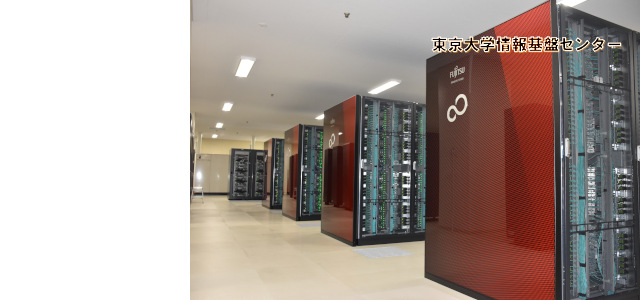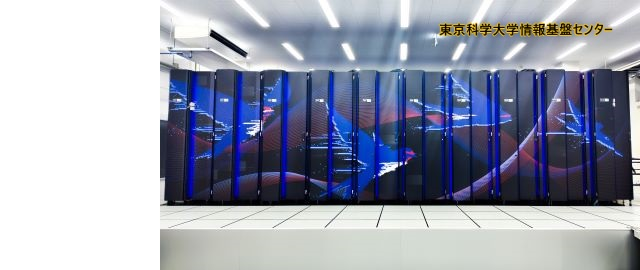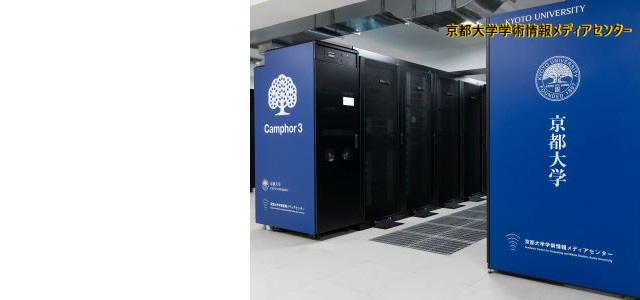•••••••••# 採択課題 【詳細】

jh200008-NAH Developing Accuracy Assured High Performance Numerical Libraries for Eigenproblems 片桐孝洋(名古屋大学・情報基盤センター) TAKAHIRO KATAGIRI (Information Technology Center, Nagoya University) Eigenproblem is one of essential numerical problems for several numerical simulations. Its accuracy, however, is not well-assured in many conventional numerical computations. Basic Linear Algebra Subprograms (BLAS) is a frequently used to perform linear algebra computations. Ensuring the accuracy of the computational results of BLAS operations is a still crucial problem now. Even in solving linear equations using LAPACK is also a typical example, because LAPACK is rich in BLAS operations, especially matrix-matrix multiplication (MMM) operations for solving linear equations. With respect to this background, we focus on the following three topics: (1) Developing an accuracy assured numerical libraries for eigenproblems; (2) Development of high-performance implementation and auto-tuning (AT) technology for the developed accuracy assured numerical libraries; (3) Discussing an extension for non-liner problems based on obtained knowledge of accuracy assured algorithms. 研究紹介ポスター ／ 最終報告書